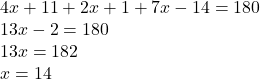## In ΔPQR, \text{m}\angle P = (4x+11)^{\circ}m∠P=(4x+11) ∘ , \text{m}\angle Q = (2x+1)^{\circ}m∠Q=(2x+1) ∘ , and \text{m}\angle R = (7x-14)^{\

Question

In ΔPQR, \text{m}\angle P = (4x+11)^{\circ}m∠P=(4x+11) ∘ , \text{m}\angle Q = (2x+1)^{\circ}m∠Q=(2x+1) ∘ , and \text{m}\angle R = (7x-14)^{\circ}m∠R=(7x−14) ∘ . Find \text{m}\angle P.m∠P.

in progress 0
2 months 2021-08-19T05:33:47+00:00 1 Answers 4 views 0

67°

Step-by-step explanation:

According to angle sum property of a triangle, sum of all the angles of a triangle is equal to 180°.

In ΔPQR,

∠P + ∠Q + ∠R = 180°

Put ∠P = (4x+11)°, ∠Q = (2x+1)° and ∠R = (7x-14)°So,

m∠P = [4(14)+11]° = 67°